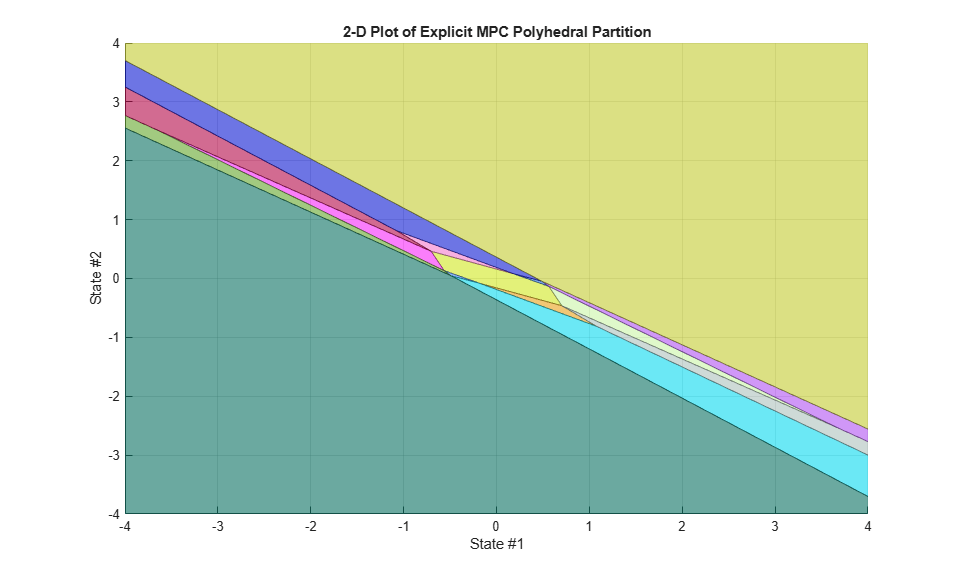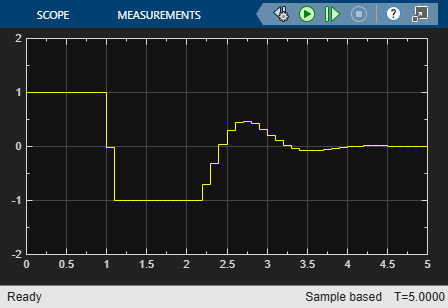Explicit MPC Control of a Single-Input-Single-Output Plant

This example shows how to control a double integrator plant under input saturation in Simulink® using explicit MPC.

For an example that controls a double integrator with an implicit MPC controller, see Model Predictive Control of a Single-Input-Single-Output Plant.

Define Plant Model

The linear open-loop dynamic model is a double integrator.

plant = tf(1,[1 0 0]);

Design MPC Controller

Create the controller object with a sample period of 0.1 seconds, and prediction and control horizons of 10 and 3 steps respectively.

Ts = 0.1;
mpcobj = mpc(plant, Ts, 10, 3);
-->The "Weights.ManipulatedVariables" property of "mpc" object is empty. Assuming default 0.00000.
-->The "Weights.ManipulatedVariablesRate" property of "mpc" object is empty. Assuming default 0.10000.
-->The "Weights.OutputVariables" property of "mpc" object is empty. Assuming default 1.00000.

Specify actuator saturation limits as manipulated variable constraints.

mpcobj.MV = struct('Min',-1,'Max',1);

Generate Explicit MPC Controller

Explicit MPC executes the equivalent explicit piecewise affine version of the MPC control law defined by traditional implicit MPC. To generate an explicit MPC object from a traditional MPC one, you must specify the range for each controller state, reference signal, manipulated variable and measured disturbance. This allows for the multi-parametric quadratic programming problem associated to the MPC problem to be set up and solved, once for all, in the parameter space defined by these ranges.

Use the generateExplicitRange function to obtain a range structure where you can specify parameter ranges.

range = generateExplicitRange(mpcobj);
-->Converting the "Model.Plant" property of "mpc" object to state-space.
-->Converting model to discrete time.
Assuming no disturbance added to measured output channel #1.
-->The "Model.Noise" property of the "mpc" object is empty. Assuming white noise on each measured output channel.

MPC controller states include states from plant model, disturbance model and noise model, in that order. Setting the range of a state variable is sometimes difficult when the state does not correspond to a physical parameter. In that case, multiple runs of open-loop plant simulation with typical reference and disturbance signals are recommended in order to collect data that reflect the ranges of states.

range.State.Min(:) = [-10;-10];
range.State.Max(:) = [10;10];

Usually you know the practical range of the reference signals being used at the nominal operating point in the plant. The ranges used to generate an explicit MPC controller must be at least as large as the practical range.

range.Reference.Min = -2;
range.Reference.Max = 2;

Specify the manipulated variable ranges. If the manipulated variables are constrained, the ranges used to generate the explicit MPC controller must be at least as large as these limits.

range.ManipulatedVariable.Min = -1.1;
range.ManipulatedVariable.Max = 1.1;

Use generateExplicitMPC command to obtain an explicit MPC controller with the specified parameter ranges.

mpcobjExplicit = generateExplicitMPC(mpcobj, range)

Regions found / unexplored:       19/       0

Explicit MPC Controller
---------------------------------------------
Controller sample time:    0.1 (seconds)
Polyhedral regions:        19
Number of parameters:      4
Is solution simplified:    No
State Estimation:          Default Kalman gain
---------------------------------------------
Type 'mpcobjExplicit.MPC' for the original implicit MPC design.
Type 'mpcobjExplicit.Range' for the valid range of parameters.
Type 'mpcobjExplicit.OptimizationOptions' for the options used in multi-parametric QP computation.
Type 'mpcobjExplicit.PiecewiseAffineSolution' for regions and gain in each solution.

Use the simplify function with the 'exact' method to join pairs of regions whose corresponding gains are the same and whose union is a convex set. Doing so can reduce memory footprint of the explicit MPC controller without sacrificing any performance.

mpcobjExplicitSimplified = simplify(mpcobjExplicit, 'exact')

Regions to analyze:       15/      15

Explicit MPC Controller
---------------------------------------------
Controller sample time:    0.1 (seconds)
Polyhedral regions:        15
Number of parameters:      4
Is solution simplified:    Yes
State Estimation:          Default Kalman gain
---------------------------------------------
Type 'mpcobjExplicitSimplified.MPC' for the original implicit MPC design.
Type 'mpcobjExplicitSimplified.Range' for the valid range of parameters.
Type 'mpcobjExplicitSimplified.OptimizationOptions' for the options used in multi-parametric QP computation.
Type 'mpcobjExplicitSimplified.PiecewiseAffineSolution' for regions and gain in each solution.

The number of piecewise affine regions has been reduced.

Plot Piecewise Affine Partition

You can review any 2-D section of the piecewise affine partition defined by the explicit MPC control law.

Use generatePlotParameters command to obtain a parameter structure where you can specify which 2-D section to plot afterwards.

params = generatePlotParameters(mpcobjExplicitSimplified)
params =

struct with fields:

State: [1x1 struct]
Reference: [1x1 struct]
MeasuredDisturbance: [1x1 struct]
ManipulatedVariable: [1x1 struct]

All but two of the control laws free parameters must be fixed, the remaining two variables form the plot axes. In this example, you plot the first state variable against the second state variable, so you need to fix all the other parameters at a value within their respective ranges.

Leave the states free to vary.

params.State.Index = [];
params.State.Value = [];

Fix the reference signal.

params.Reference.Index = 1;
params.Reference.Value = 0;

Fix the manipulated variable.

params.ManipulatedVariable.Index = 1;
params.ManipulatedVariable.Value = 0;

Use plotSection command to plot the 2-D section defined by the two free parameters. For more information, see plotSection.

plotSection(mpcobjExplicitSimplified, params);
axis([-4 4 -4 4]);
grid
xlabel('State #1');
ylabel('State #2');Simulate Using mpcmove Function

Compare closed-loop simulations for traditional implicit MPC and explicit MPC using the mpcmove and mpcmoveExplicit functions respectively.

Initialize variables to store the closed-loop MPC responses.

Tf = round(5/Ts);
YY = zeros(Tf,1);
YYExplicit = zeros(Tf,1);
UU = zeros(Tf,1);
UUExplicit = zeros(Tf,1);

Prepare the plant model used in simulation

sys = c2d(ss(plant),Ts);
xsys = [0;0];
xsysExplicit = xsys;

To obtain a pointer to the internal states for both controllers, use mpcstate.

xmpc = mpcstate(mpcobj);
xmpcExplicit = mpcstate(mpcobjExplicitSimplified);

Iteratively simulate the closed-loop response for both controllers.

for t = 0:Tf

% update plant measurement
ysys = sys.C*xsys;
ysysExplicit = sys.C*xsysExplicit;

u = mpcmove(mpcobj,xmpc,ysys,1);
% compute explicit MPC action
uExplicit = mpcmoveExplicit(mpcobjExplicit,xmpcExplicit,ysysExplicit,1);

% store signals
YY(t+1)=ysys;
YYExplicit(t+1)=ysysExplicit;
UU(t+1)=u;
UUExplicit(t+1)=uExplicit;

% update plant state
xsys = sys.A*xsys + sys.B*u;
xsysExplicit = sys.A*xsysExplicit + sys.B*uExplicit;
end

% Norm of the differences between traditional and explicit controller signals.
fprintf('\nDifference between traditional and Explicit MPC responses using MPCMOVE command is %g\n',...
norm(UU-UUExplicit)+norm(YY-YYExplicit));
Difference between traditional and Explicit MPC responses using MPCMOVE command is 2.75324e-13

Simulate Using sim Function

Compare closed-loop simulations between traditional and explicit MPC using the sim command.

Tf = 5/Ts;                      % number of simulation iterations
[y1,t1,u1] = sim(mpcobj,Tf,1);  % simulate with traditional MPC
[y2,t2,u2] = sim(mpcobjExplicitSimplified,Tf,1); % simulate with explicit MPC
-->Converting the "Model.Plant" property of "mpc" object to state-space.
-->Converting model to discrete time.
Assuming no disturbance added to measured output channel #1.
-->The "Model.Noise" property of the "mpc" object is empty. Assuming white noise on each measured output channel.

The simulation results are identical.

fprintf('\nDifference between traditional and Explicit MPC responses using SIM command is %g\n',...
norm(u2-u1)+norm(y2-y1));
Difference between traditional and Explicit MPC responses using SIM command is 2.75705e-13

Simulate the traditional MPC controller in Simulink. The MPC Controller block is configured to use mpcobj as its controller.

mdl = 'mpc_doubleint';
open_system(mdl)
sim(mdl)Simulate the explicit MPC controller in Simulink. The Explicit MPC Controller block is configured to use mpcobjExplicitSimplified as its controller.

mdlExplicit = 'empc_doubleint';
open_system(mdlExplicit)
sim(mdlExplicit)The closed-loop responses are identical.

norm(uExplicit-u)+norm(yExplicit-y));